• Python 绘制 柱状图
千次阅读
2020-11-20 19:21:39

#创建一个点数为 8 x 6 的窗口, 并设置分辨率为 80像素/每英寸

plt.figure(figsize=(10, 10), dpi=80)#再创建一个规格为 1 x 1 的子图#plt.subplot(1, 1, 1)#柱子总数

N = 10

#包含每个柱子对应值的序列

values = (56796,42996,24872,13849,8609,5331,1971,554,169,26)#包含每个柱子下标的序列

index =np.arange(N)#柱子的宽度

width = 0.45

#绘制柱状图, 每根柱子的颜色为紫罗兰色

p2 = plt.bar(index, values, width, label="num", color="#87CEFA")#设置横轴标签

plt.xlabel('clusters')#设置纵轴标签

plt.ylabel('number of reviews')#添加标题

plt.title('Cluster Distribution')#添加纵横轴的刻度

plt.xticks(index, ('mentioned1cluster', 'mentioned2cluster', 'mentioned3cluster', 'mentioned4cluster', 'mentioned5cluster', 'mentioned6cluster', 'mentioned7cluster', 'mentioned8cluster', 'mentioned9cluster', 'mentioned10cluster'))#plt.yticks(np.arange(0, 10000, 10))#添加图例

plt.legend(loc="upper right")

plt.show()

更多相关内容
• ## python绘制柱状图

万次阅读 多人点赞 2019-07-02 20:53:31
使用 Matplotlib 提供的bar()函数来绘制柱状图。与前面介绍的 plot() 函数类似，程序每次调用 bar() 函数时都会生成一组柱状图， 如果希望生成多组柱状图，则可通过多次调用 bar() 函数来实现。 下面程序使用柱状图...

使用 Matplotlib 提供的 bar() 函数来绘制柱状图。与前面介绍的 plot() 函数类似，程序每次调用 bar() 函数时都会生成一组柱状图， 如果希望生成多组柱状图，则可通过多次调用 bar() 函数来实现。

下面程序使用柱状图来展示《C语言基础》和《Java基础》两套教程历年的销量数据。

import matplotlib.pyplot as plt
import numpy as np

# 构建数据
x_data = ['2012', '2013', '2014', '2015', '2016', '2017', '2018']
y_data = [58000, 60200, 63000, 71000, 84000, 90500, 107000]
y_data2 = [52000, 54200, 51500,58300, 56800, 59500, 62700]
# 绘图
plt.bar(x=x_data, height=y_data, label='C语言基础', color='steelblue', alpha=0.8)
plt.bar(x=x_data, height=y_data2, label='Java基础', color='indianred', alpha=0.8)
# 在柱状图上显示具体数值, ha参数控制水平对齐方式, va控制垂直对齐方式
for x, y in enumerate(y_data):
plt.text(x, y + 100, '%s' % y, ha='center', va='bottom')
for x, y in enumerate(y_data2):
plt.text(x, y + 100, '%s' % y, ha='center', va='top')
# 设置标题
plt.title("Java与Android图书对比")
# 为两条坐标轴设置名称
plt.xlabel("年份")
plt.ylabel("销量")
# 显示图例
plt.legend()
plt.show()

上面程序中，第 9、10 两行代码用于在数据图上生成两组柱状图，程序设置了这两组柱状图的颜色和透明度。

在使用 bar() 函数绘制柱状图时，默认不会在柱状图上显示具体的数值。为了能在柱状图上显示具体的数值，程序可以调用 text() 函数在数据图上输出文字，如上面程序中第 10 行代码所示。

在使用 text() 函数输出文字时，该函数的前两个参数控制输出文字的 X、Y 坐标，第三个参数则控制输出的内容。其中 va 参数控制文字的垂直对齐方式，ha 参数控制文字的水平对齐方式。

对于上面的程序来说，由于 X 轴数据是一个字符串列表，因此 X 轴实际上是以列表元素的索引作为刻度值的。因此，当程序指定输出文字的 X 坐标为 0 时，表明将该文字输出到第一个条柱处；对于 Y 坐标而言，条柱的数值正好在条柱高度所在处，如果指定 Y 坐标为条柱的数值 +100，就是控制将文字输出到条柱略上一点的位置。

运行上面程序，可以看到如图 1 所示的效果。图 1 两组柱状图

从图 1 所示的显示效果来看，第二次绘制的性状图完全与第一次绘制的柱状图重叠，这并不是我们期望的结果，我们希望每组数据的条柱能并列显示。

为了实现条柱井列显示的效果，首先分析条柱重叠在一起的原因。使用 Matplotlib 绘制柱状图时同样也需要 X 轴数据，本程序的 X 轴数据是元素为字符串的 list 列表，因此程序实际上使用各字符串的索引作为 X 轴数据。比如 '2011' 字符串位于列表的第一个位置，因此代表该条柱的数据就被绘制在 X 轴的刻度值1处（由于两个柱状图使用了相同的 X 轴数据，因此它们的条柱完全重合在一起）。

为了将多个柱状图的条柱并列显示，程序需要为这些柱状图重新计算不同的 X 轴数据。为了精确控制条柱的宽度，程序可以在调用 bar() 函数时传入 width 参数，这样可以更好地计算条柱的并列方式。将上面程序改为如下形式：

import matplotlib.pyplot as plt
import numpy as np

# 构建数据
x_data = ['2011', '2012', '2013', '2014', '2015', '2016', '2017']
y_data = [58000, 60200, 63000, 71000, 84000, 90500, 107000]
y_data2 = [52000, 54200, 51500,58300, 56800, 59500, 62700]
bar_width=0.3
# 将X轴数据改为使用range(len(x_data), 就是0、1、2...
plt.bar(x=range(len(x_data)), height=y_data, label='C语言基础',
color='steelblue', alpha=0.8, width=bar_width)
# 将X轴数据改为使用np.arange(len(x_data))+bar_width,
# 就是bar_width、1+bar_width、2+bar_width...这样就和第一个柱状图并列了
plt.bar(x=np.arange(len(x_data))+bar_width, height=y_data2,
label='Java基础', color='indianred', alpha=0.8, width=bar_width)
# 在柱状图上显示具体数值, ha参数控制水平对齐方式, va控制垂直对齐方式
for x, y in enumerate(y_data):
plt.text(x, y + 100, '%s' % y, ha='center', va='bottom')
for x, y in enumerate(y_data2):
plt.text(x+bar_width, y + 100, '%s' % y, ha='center', va='top')
# 设置标题
plt.title("C与Java对比")
# 为两条坐标轴设置名称
plt.xlabel("年份")
plt.ylabel("销量")
# 显示图例
plt.legend()
plt.show()

该程序与前一个程序的区别就在于第 10、14 两行代码，这两行代码使用了不同的 x 参数，其中第一个柱状图的 X 轴数据为 range(len(x_data))，也就是 0、1、2…，这样第一个柱状图的各条柱恰好位于 0、1、2… 刻度值处；第二个柱状图的 X 轴数据为 np.arange(len(x_data))+bar_width，也就是 bar_width、1+bar_width、2+bar_width···，这样第二个柱状图的各条柱位于 0、1、2…刻度值的偏右一点 bar_width 处，这样就恰好与第一个柱状图的各条柱并列了。

运行上面程序，将会发现该柱状图的 X 轴的刻度值变成 0、1、2 等值，不再显示年份。为了让柱状图的 X 轴的刻度值显示年份，程序可以调用 xticks() 函数重新设置 X 轴的刻度值。

例如，在程序中添加如下代码：

# 为X轴设置刻度值
plt.xticks(np.arange(len(x_data))+bar_width/2, x_data)

上面代码使用 x_data 为 X 轴设置刻度值，第一个参数用于控制各刻度值的位置，该参数是 np.arange(len(x_data))+bar_width/2，也就是 bar_width/2、1+bar_width/2、2+bar_width/2 等，这样这些刻度值将被恰好添加在两个条柱之间。

运行上面程序可看到如图 2 所示的运行结果：图 2 并列的柱状图

有些时候，可能希望两个条柱之间有一点缝隙，那么程序只要对第二个条柱的 X 轴数据略做修改即可。例如，将上面程序中第 14 行代码改为如下形式：

plt.bar(x=np.arange(len(x_data))+bar_width+0.05, height=y_data2,
label='Java基础', color='indianred', alpha=0.8, width=bar_width)

上面代码重新计算了 X 轴数据，使用 np.arange(len(x_data))+bar_width+0.05 作为 X 轴数据，因此两组柱状图的条柱之间会有 0.05 的距离。

## Matplotlib 绘制水平柱状图

调用 Matplotlib 的 barh() 函数可以生成水平柱状图。barh() 函数的用法与 bar() 函数的用法基本一样，只是在调用 barh() 函数时使用 y参数传入 Y 轴数据，使用 width 参数传入代表条柱宽度的数据。

例如，如下程序调用 barh() 函数生成两组并列的水平柱状图，来展示两套教程历年的销量统计数据：

import matplotlib.pyplot as plt
import numpy as np

# 构建数据
x_data = ['2011', '2012', '2013', '2014', '2015', '2016', '2017']
y_data = [58000, 60200, 63000, 71000, 84000, 90500, 107000]
y_data2 = [52000, 54200, 51500,58300, 56800, 59500, 62700]
bar_width=0.3
# Y轴数据使用range(len(x_data), 就是0、1、2...
plt.barh(y=range(len(x_data)), width=y_data, label='Java基础教程',
color='steelblue', alpha=0.8, height=bar_width)
# Y轴数据使用np.arange(len(x_data))+bar_width,
# 就是bar_width、1+bar_width、2+bar_width...这样就和第一个柱状图并列了
plt.barh(y=np.arange(len(x_data))+bar_width, width=y_data2,
label='C语言基础', color='indianred', alpha=0.8, height=bar_width)

# 在柱状图上显示具体数值, ha参数控制水平对齐方式, va控制垂直对齐方式
for y, x in enumerate(y_data):
plt.text(x+5000, y-bar_width/2, '%s' % x, ha='center', va='bottom')
for y, x in enumerate(y_data2):
plt.text(x+5000, y+bar_width/2, '%s' % x, ha='center', va='bottom')
# 为Y轴设置刻度值
plt.yticks(np.arange(len(x_data))+bar_width/2, x_data)
# 设置标题
plt.title("Java与C对比")
# 为两条坐标轴设置名称
plt.xlabel("销量")
plt.ylabel("年份")
# 显示图例
plt.legend()
plt.show()

上面程序中，第 10 行代码使用 barh() 函数来创建水平柱状图，其中 y 参数为 range(len(x_data))，这意味着这些条柱将会沿着 Y 轴均匀分布；而 width 参数为 y_data，这意味着 y_data 列表所包含的数值会决定各条柱的宽度。第 14 行代码的控制方式与此类似。

运行上面程序，可以看到如图 3 所示的效果。图 3 水平柱状图

reference:

http://c.biancheng.net/view/2716.html

展开全文• 主要介绍了Python实现绘制柱状图并显示数值功能,涉及Python数值运算及基于matplotlib的图形绘制相关操作技巧,需要的朋友可以参考下
• python绘制柱状图

# Ⅰ.原始数据

2022北京冬奥会奖牌榜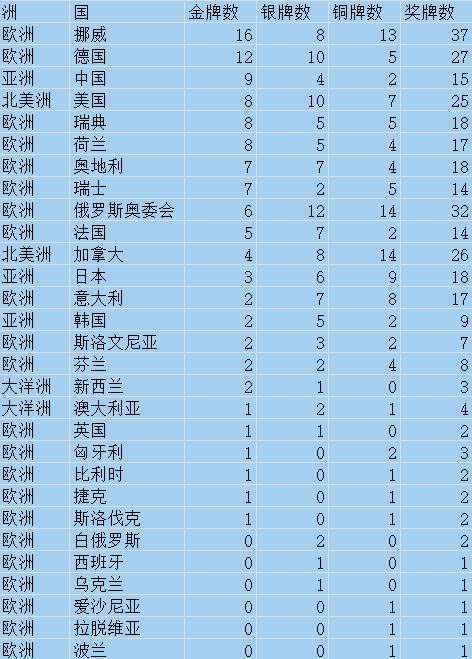# Ⅱ.程序

from pyecharts import options as opts
from pyecharts.charts import Bar

c=(
Bar()
.set_global_opts(
xaxis_opts=opts.AxisOpts(axislabel_opts=opts.LabelOpts(rotate=-30)),
title_opts=opts.TitleOpts(title='2022北京冬奥会奖牌榜',subtitle='2022/02/04-2022/02/20'),
toolbox_opts=opts.ToolboxOpts(),
datazoom_opts=opts.DataZoomOpts()
)
.render('map.html')
)

# Ⅲ.效果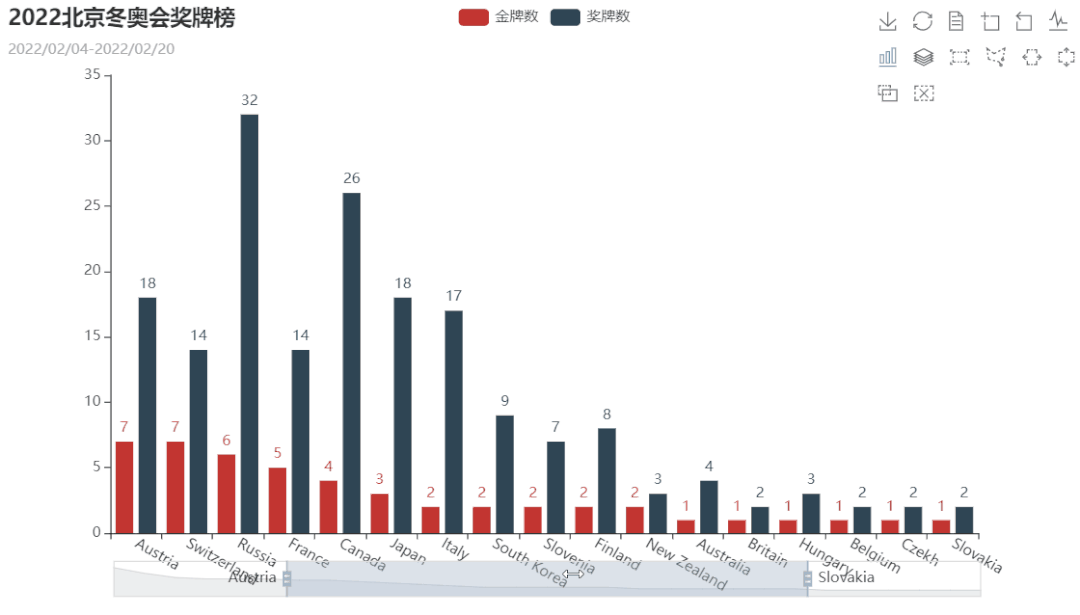展开全文echarts
• 利用python可以对数据进行可视化分析，这里展示两种常见的图形，分别是柱状图和折线图。 欢迎大家转发，一起传播知识和正能量，帮助到更多人。辛苦大家转发时注明出处(也是咱们公益编程交流群的入口网址)，刘...

利用python可以对数据进行可视化分析，这里展示两种常见的图形，分别是柱状图和折线图。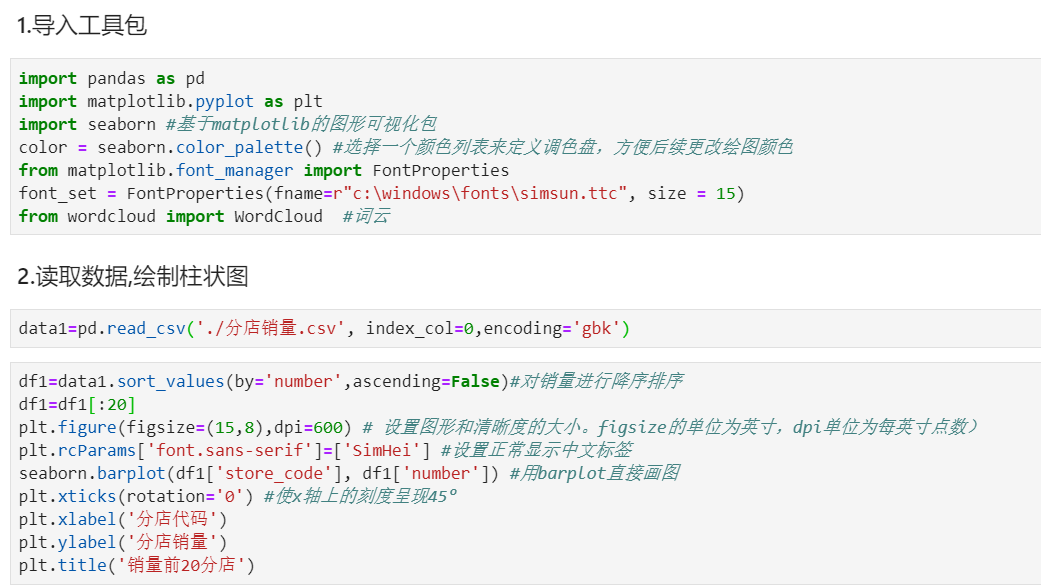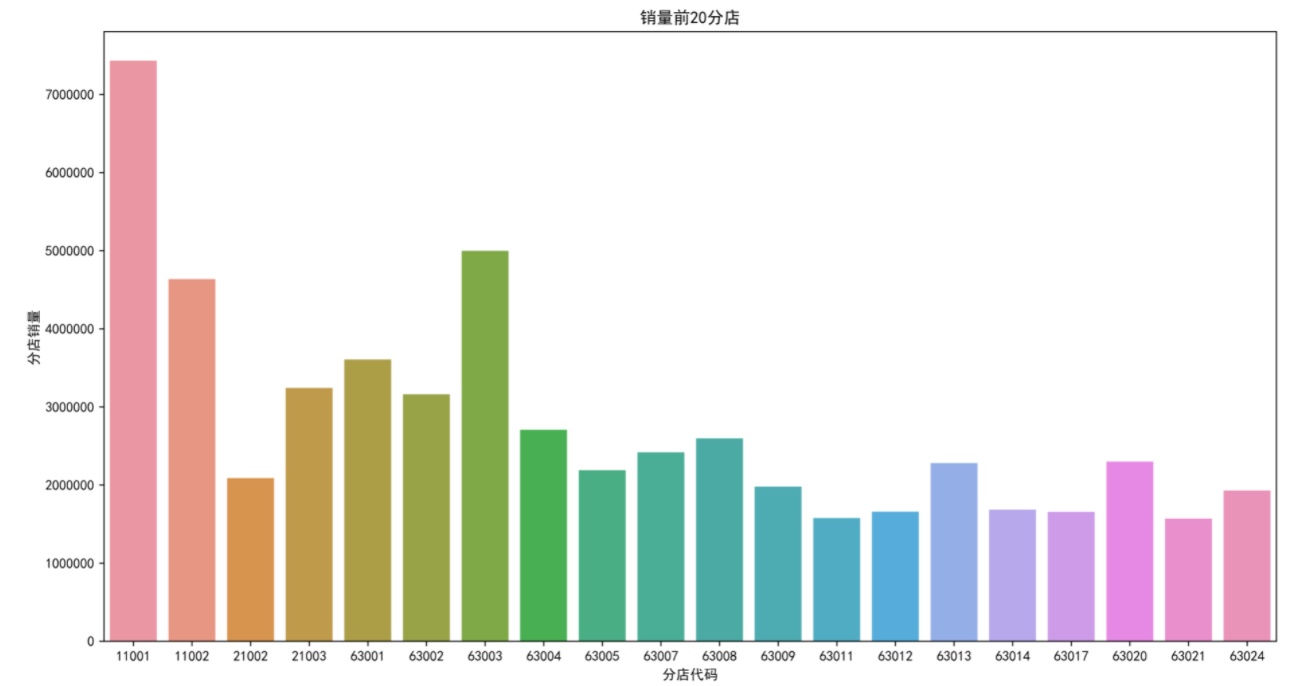欢迎大家转发，一起传播知识和正能量，帮助到更多人。辛苦大家转发时注明出处(也是咱们公益编程交流群的入口网址)，刘经纬老师共享知识相关文件下载地址为：http://liujingwei.cn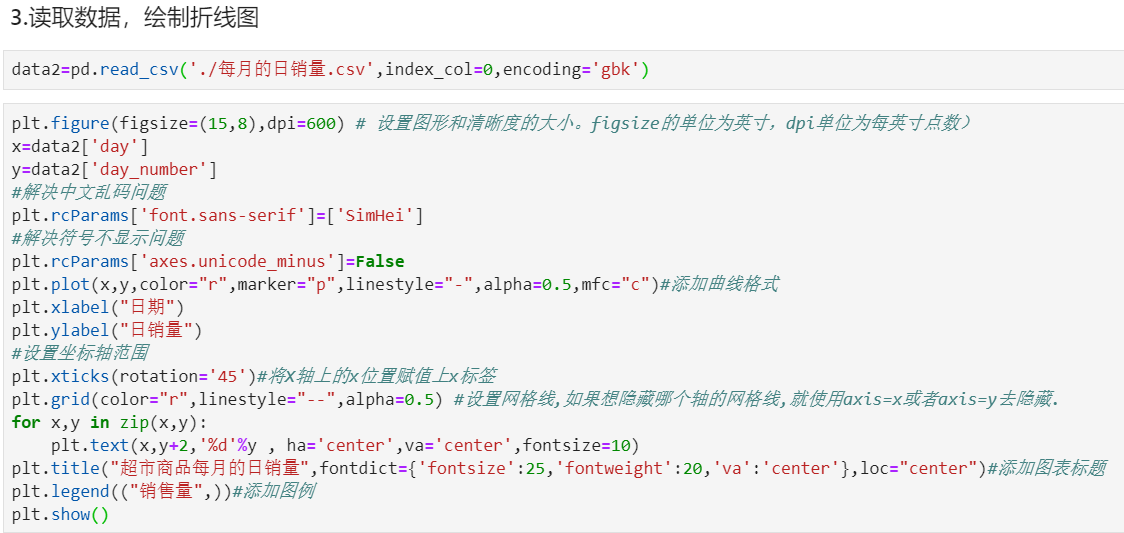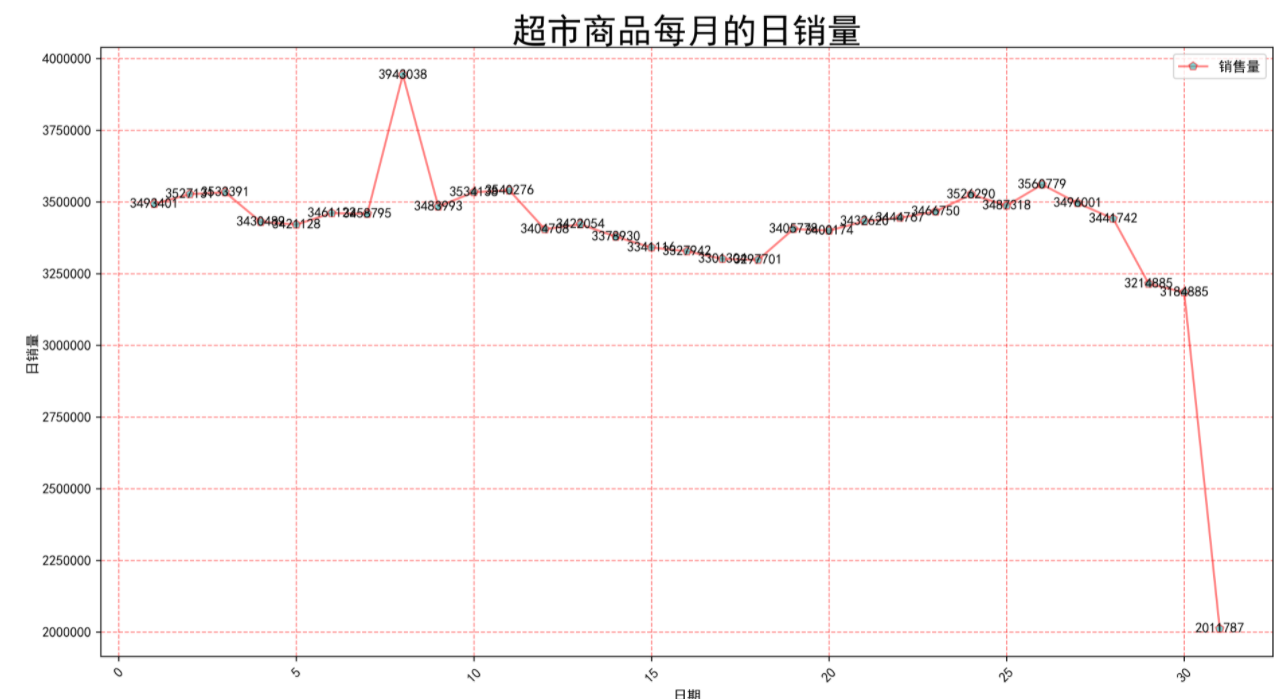展开全文• python用matplotlib画图时不显示中文，显示乱码小正方形 在程序最前面加上，如下代码 # encoding=utf-8 import matplotlib.pyplot as plt ...Python绘制柱状图并显示数值 # encoding=utf-8 import
• 今天小编就为大家分享一篇python柱状图--不同颜色并显示数值的方法，具有很好的参考价值，希望对大家有所帮助。一起跟随小编过来看看吧
• #若是不想显示直线，可以直接将上面两行注释掉 plt.bar(index, pvalue, width,color="#87CEFA") #绘制柱状图 #plt.xlabel('cell type') #x轴 plt.ylabel('p value') #y轴 plt.title('Result of Network Screen') #...
• 在使用Python绘制图表前，我们需要先安装两个库文件numpy和matplotlib。Numpy是Python开源的数值计算扩展，可用来存储和处理大型矩阵，比Python自身数据结构要高效；matplotlib是一个Python的图像框架，使用其绘制...
• #若是不想显示直线，可以直接将上面两行注释掉 plt.bar(index, pvalue, width,color="#87CEFA") #绘制柱状图 #plt.xlabel('cell type') #x轴 plt.ylabel('p value') #y轴 plt.title('Result of Network Screen') #...
• 对《青春有你2》对选手体重及地区分布进行可视化...方法一：maplotlib方式绘制：plt.bar函数生成柱状图 import numpy as np import json import matplotlib.font_manager as font_manager #显示matplotlib生成的图...数据可视化
• 首先，详细探讨如何用Python绘制柱状图柱状图是大家最为熟悉的一类图表了，在咱们的平常工作中也是使用频率非常高的一种统计图形哦，下面咱们还是结合“栗子”的方式对其进行详细的聊聊哦！举个“栗子”哦，然后结合...
• Python绘制柱状图之可视化神器Pyecharts 昨晚写了关于Matplotlib的可视化，今天推荐一个可视化的利器-pyecharts。 前文链接：Python可视化图表生成-Matplotlib 安装 pip install -i ...
• 根据步骤二得到的实验数据，绘制柱状图、条形图 练习如何通过调整参数使图片呈现不同效果，例如颜色、图例位置、背景网格、坐标轴刻度和标记等 实验过程（附结果截图）： 1. 练习python中柱状图、条形图绘图函数的...数据可视化
• 本文的文字及图片来源于网络,仅供学习、交流使用,不具有任何商业用途,版权归原作者所有,如有问题请及时联系我们以作处理以下文章来源于数据Magic，作者我不是小样前言python里面有很多优秀的可视化库，matplotlib，...
• plt.barh()表示绘制水平方向的柱状图，plt.bar()表示绘制竖直方向的柱状图。plt.scatter表示绘制散点图，plt.pie()表示绘制饼状图。 [太阳]选择题 以下哪一种方法可以画竖向柱状图？ A bar() B barh() C ...c语言 java
• 我们经常看到的Bar Chart Race（柱形竞赛），可以看到数据的呈现...1、导入PythonPython12345importpandasaspdimportmatplotlib.pyplotaspltimportmatplotlib.tickerastickerimportmatplotlib.animationasanimat...
• 一、使用plot()方法绘制柱状图 二、查看DataFrame的内存占用情况 三、concat()函数与append()方法 四、merge()方法与join()方法 以及里面含有如何区分loc与iloc等细节问题：
• import matplotlib.pyplot as plt #设置中文字体 plt.rcParams['font.family'] = ['SimHei'] x1 = [1,3,5,7,9] y1 = [8,6,2,5,6] x2 = [2,4,6,8,10] ...plt.title('绘制柱状图') plt.ylabel('y轴') plt.数据可视化 深度学习
• 小编之前也和大家分享过python使用matplotlib实现的折线图和制饼图效果，感兴趣的朋友们也可以点击查看，下面来看看python使用matplotlib绘制柱状图的方法吧，具体如下： 1. 基本的柱状图 import matplotlib.pyplot...
• 对比excel，用python绘制柱状图时添加table数据表.pdf
• 原标题：零基础用 Python 画图表，让你的论文高一个逼格曾经我在高中的时候有一篇数学探索的小作业，其中我需要画出一张比较复杂的函数。由于 X 和 Y 的坐标轴标签都需要一定的自定义，所以微软 Office 自带的画图...
• 本文主要讲解这四个大方面...一、使用plot()方法绘制柱状图等其它图形 二、查看DataFrame的内存占用情况 三、concat()函数与append()方法 四、merge()方法与join()方法 项目的结构为： 代码字体以及Excel到这里面取： ...开发语言 后端
• 1、插件安装 安装两种插件 pip install -i ... 2、普通柱状图 柱状图绘制步骤 导入matplotlib.pyplot模块 准备数据，可以使用numpy/pandas整理数据 调用pyplot.bar()绘制柱状图 上代码 import numpy as np impmatplotlib
• import matplotlib.pyplot as plt import matplotlib as mpl import numpy as np mpl.rcParams["font.sans-serif"]=["SimHei"]#显示中文 name=["土豆","地瓜","西红柿"] weight=[70,80,60] plt.bar(name,weight) ...
• 主要介绍了Python 堆叠柱状图绘制方法，文中通过示例代码介绍的非常详细，对大家的学习或者工作具有一定的参考学习价值，需要的朋友们下面随着小编来一起学习学习吧...